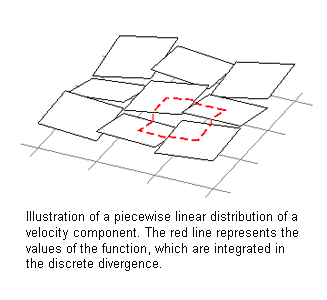Springe direkt zu Inhalt

# Computational Methods for Weakly Compressible FlowsWeakly compressible flows typically arise in geophysical applications, namely in atmospheric and oceanic flows, but also they are of interest for combustion problems. Mathematically, these problems are described by the Euler and Navier-Stokes equations for the conservation of mass, momentum and energy. They are characterized (in their non-dimensional form) by small Mach-, Froude-, Rossby- and Reynolds numbers. Specifically, the Mach number, which represents the ratio between the typical flow velocity of the problem and the speed of sound, describes the influence of compressibility in a given flow problem.

Under certain conditions the shallow water equations are a good approximation describing the temporal evolution of flows with a small vertical extend compared to their horizontal dimension. In each horizontal point, the equations determine the temporal change of the flow depth and as well as the flow velocity averaged over the depth of the fluid. in certain problems, velocity of surface waves becomes large to the fluid velocity itself. This is mathematically described by a small Froude number, and it is equivalent to the appearance to a small Mach number in the compressible fluid equations. A prominent example for shallow water flows with small Froude number are Tsunami waves, which propagate over whole ocean basins. However, when a Tsunami wave reaches the beach, the shallow water theory becomes very inaccurate.

In the construction of numerical methods for the solution of such weakly compressible flows, one is faced with the problem that the equations are singular in the limit of vanishing Mach resp. Froude numbers; i.e. they change their type from hyperbolic to hyperbolic-elliptic. As a consequence, spatial pressure variations vanish in the limit, but they do affect the velocity field at leading order. Furthermore, this behavior implies a divergence constraint on the mass flux. If one is also interested in an explicit method, where the solution at a grid point is determined from the solution of earlier time steps in the vicinity of this point, one can only perform very small time steps due to the large sound speed. Otherwise, the method is not stable, and it might not approximate the physical wave propagation well. In atmospheric applications, on the other hand, one is usually not interested in sound waves, but in the general flow, which is mostly not influenced by compressibility effects. Thus, one also likes to perform "large" time steps which are only dictated by the slow convective flow.

Our approach for the numerical solution of weakly compressible flows is based on so called projection methods, which can be used for the solution of the incompressible limit equations, i.e., in the limit of a vanishing Mach resp. Froude number. In these methods, the (slightly modified) equations are solved without considering the divergence constraint in a first step. This is done by the application of Godunov-type methods for hyperbolic conservation laws. In a second step, the fluxes determined in the first step are corrected by two projections such that the arising velocities satisfy the above mentioned divergence constraint. Our group develops such methods for the application in combustion and geophysical problems, analyzes their stability and convergence properties, and works on techniques for the representation of complex boundary geometries.

The reader is referred to the work of Schneider et al. (1999) and Vater (2005) concerning the formulation of the projection method, to Vater and Klein (2009) concerning the stability of the projection, to Kadioglu et al. (2009) about the extension of the method to forth order, and to Klein (2009) concerning its application to "sound-proof" models. Current research is focused on the extension of the described technology from the application to incompressible to weakly compressible flows with small but non-zero Mach resp. Froude numbers. Earlier attempts were successfully implemented by Klein (1995) for one space dimension and by Geratz (1997) and Munz et al. (2003) for multiple space dimensions. It is planned that the ideas proposed in these references are extended by the combination of results from multiple scale analysis and concepts of multigrid techniques.